# Quiz 9: Absorption Cost System

Conversion Costs: It is the sum of all the direct costs incurred during the process of manufacturing and all the overhead costs which are incurred during such a process. FIFO: It stands for first in first out and it is a method of recording the inventory by the company that the oldest inventory is to sell first by the company. In the present case, the data regarding the working of the firm in the first quarter of the year are provided. Before calculating the requirement of the given case, one should understand the relation between ending the beginning work in process and the same is presented with the help of equations which are as follows: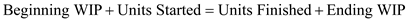Now, compute the conversion with the help of MS-Excel which is as follows: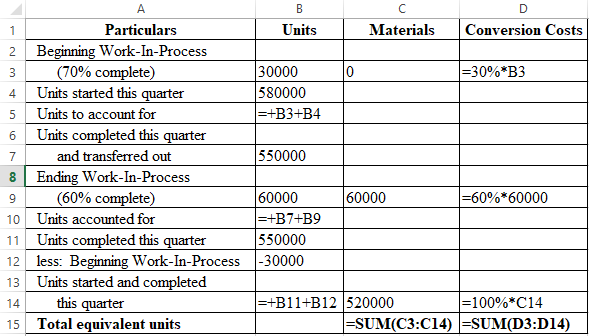The result of the above calculations is as follows: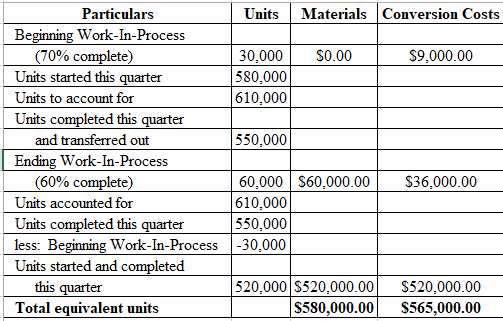Therefore the conversion costs come out to be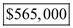Budgeted volume: Budgeted volume means the quantity planned either to produce or sell for a given particular period. Volume expected for the coming year or the long run average volumes are the two ways to estimate the budgeted volume. Calculate the budgeted volume amount (in screen square inches) as shown below: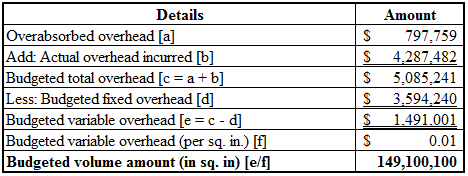The over applied refers to excess absorption of overheads. Therefore the actual overheads are less than the applied overheads. Therefore, to get the budgeted overheads the over applied should be added to the actual overheads. Thus, the budgeted volume amount (in sq. in) is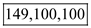.